# OpenCV Line Detection

In this tutorial, let’s learn how to use Hough line transformation with OpenCV to make line detection in an Image.

## Hough Line Transform

The Hough Line Transform is a transform used to detect straight lines. OpenCV implements three kinds of Hough Line Transforms:(Standard Hough Transform, SHT),（Multi-Scale Hough Transform, MSHT）and (Progressive Probabilistic Hough Transform, PPHT).

## Theory

In the Cartesian coordinate system, the line can be expressed as y = mx+b. In general, the straight line can be represented as a point (b, m) in the parameter space.

We can express lines in the Polar system. Hence, a line equation can be written as: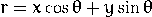where r is the length of a normal from the origin to this line and theta is the orientation of r with respect to the X-axis. For each point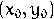, we can find a series of lines that goes through that point which means that each pair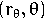represents each line that passes by. And for each point, it can be represented as a sinusoid.

It means for each point in X-Y coordinate system can be represented as a sinusoid in the r-theta coordinate system. So all the points can be described as a series of sinusoids. If the curves of two different points intersect in the plane θ – r, that means that both points belong to a same line.

So we can detect a line by finding the number of intersections between curves. In general, we can define a threshold of the minimum number of intersections needed to identify a line. For more information, please refer to http://docs.opencv.org/doc/tutorials/imgproc/imgtrans/hough_lines/hough_lines.html.

## Using Hough Line Transformation with OpenCV

### Functions for HoughLine Transform

```void HoughLines( InputArray image, OutputArray lines,

double rho, double theta, int threshold,

double srn, double stn )```
• image: Output of the edge detector. It should be a grayscale image.
• lines: A vector that will store the parameters  of the detected lines
• rho: The resolution of the parameter in pixels. We use 1 pixel.
• theta: The resolution of the parameter in radians. We use 1 degree (CV_PI/180)
• threshold: The minimum number of intersections to “detect” a line
• srn and stn: Default parameters to zero.
```void HoughLinesP( InputArray image, OutputArray lines,

double rho, double theta, int threshold,

double minLineLength=0, double maxLineGap=0 );```
• img: Output of the edge detector. It should be a grayscale image.
• lines: A vector that will store the parameters  of the detected lines
• rho: The resolution of the parameter in pixels. We use 1 pixel.
• theta: The resolution of the parameter in radians. We use 1 degree (CV_PI/180)
• threshold: The minimum number of intersections to “detect” a line
• minLinLength: The minimum number of points that can form a line. Lines with less than this number of points are disregarded.
• maxLineGap: The maximum gap between two points to be considered in the same line.

### The sample code

```#include "highgui/highgui.hpp"
#include "imgproc/imgproc.hpp"

#include <iostream>

using namespace cv;
using namespace std;

int DetectLines(const char* filename, const char* sourceName, const char* destName)
{
if (src.empty())
{
cout << "can not open " << filename << endl;
return -1;
}

Mat dst, cdst;
Canny(src, dst, 50, 200, 3);
cvtColor(dst, cdst, COLOR_GRAY2BGR);

vector<Vec4i> lines;
HoughLinesP(dst, lines, 1, CV_PI / 180, 50, 50, 10);
for (size_t i = 0; i < lines.size(); i++)
{
Vec4i l = lines[i];
line(cdst, Point(l, l), Point(l, l), Scalar(0, 0, 255), 3, 2);
}

imshow(sourceName, src);
imshow(destName, cdst);

return 0;
}

int main(int argc, char** argv)
{
DetectLines("..\\1.bmp", "line src", "line dest");
DetectLines("..\\2.jpg", "door src", "door dest");
waitKey();

return 0;
}```

### Line Detection Results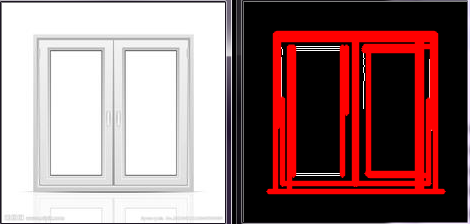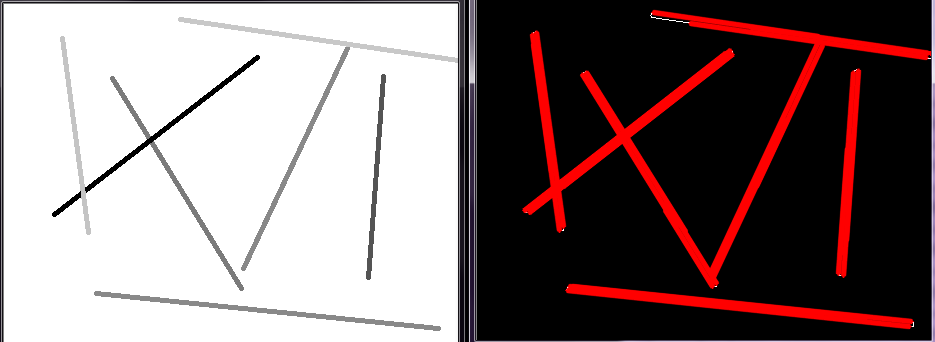## Source Code

https://github.com/DynamsoftRD/opencv-programming/tree/master/line-detection

## One thought on “OpenCV Line Detection”

1.Osman Marwat says:

Can you please tell me the java code for line detection for android